# Introduction to Vibration of Multi Degree of Freedom Systems (Part - 1) Civil Engineering (CE) Notes | EduRev

## Civil Engineering (CE) : Introduction to Vibration of Multi Degree of Freedom Systems (Part - 1) Civil Engineering (CE) Notes | EduRev

The document Introduction to Vibration of Multi Degree of Freedom Systems (Part - 1) Civil Engineering (CE) Notes | EduRev is a part of the Civil Engineering (CE) Course Introduction to Dynamics and Vibrations- Notes, Videos, MCQs.
All you need of Civil Engineering (CE) at this link: Civil Engineering (CE)

5.5 Introduction to vibration of systems with many degrees of freedom

The simple 1DOF systems analyzed in the preceding section are very helpful to develop a feel for the general characteristics of vibrating systems. They are too simple to approximate most real systems, however. Real systems have more than just one degree of freedom. Real systems are also very rarely linear. You may be feeling cheated – are the simple idealizations that you get to see in intro courses really any use? It turns out that they are, but you can only really be convinced of this if you know how to analyze more realistic problems, and see that they often behave just like the simple idealizations.

The motion of systems with many degrees of freedom, or nonlinear systems, cannot usually be described using simple formulas. Even when they can, the formulas are so long and complicated that you need a computer to evaluate them. For this reason, introductory courses typically avoid these topics. However, if you are willing to use a computer, analyzing the motion of these complex systems is actually quite straightforward – in fact, often easier than using the nasty formulas we derived for 1DOF systems.

This section of the notes is intended mostly for advanced students, who may be insulted by simplified models. If you are feeling insulted, read on…

5.5.1 Equations of motion for undamped linear systems with many degrees of freedom.

We always express the equations of motion for a system with many degrees of freedom in a standard form. The two degree of freedom system shown in the picture can be used as an example. We won’t go through the calculation in detail here (you should be able to derive it for yourself – draw a FBD, use Newton’s law and all that tedious stuff), but here is the final answer: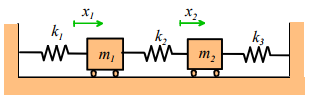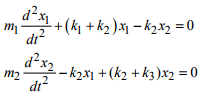To solve vibration problems, we always write the equations of motion in matrix form. For an undamped system, the matrix equation of motion always looks like this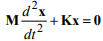where x is a vector of the variables describing the motion, M is called the ‘mass matrix’ and K is called the ‘Stiffness matrix’ for the system. For the two spring-mass example, the equation of motion can be written in matrix form as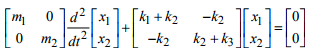For a system with two masses (or more generally, two degrees of freedom), M and K are 2x2 matrices. For a system with n degrees of freedom, they are nxn matrices.

The spring-mass system is linear. A nonlinear system has more complicated equations of motion, but these can always be arranged into the standard matrix form by assuming that the displacement of the system is small, and linearizing the equation of motion. For example, the full nonlinear equations of motion for the double pendulum shown in the figure are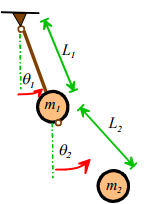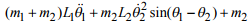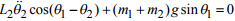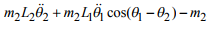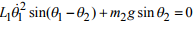Here, a single dot over a variable represents a time derivative, and a double dot represents a second time derivative (i.e. acceleration). These equations look horrible (and indeed they are – the motion of a double pendulum can even be chaotic), but if we assume that if θ1 , θ2 , and their time derivatives are all small, so that terms involving squares, or products, of these variables can all be neglected, that and recall that cos(x) ≈ 1 and sin(x) ≈ x  for small x, the equations simplify to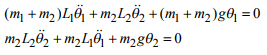Or, in matrix form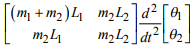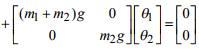This is again in the standard form.

Throughout the rest of this section, we will focus on exploring the behavior of systems of springs and masses. This is not because spring/mass systems are of any particular interest, but because they are easy to visualize, and, more importantly the equations of motion for a spring-mass system are identical to those of any linear system. This could include a realistic mechanical system, an electrical system, or anything that catches your fancy. (Then again, your fancy may tend more towards nonlinear systems, but if so, you should keep that to yourself).

5.5.2 Natural frequencies and mode shapes for undamped linear systems with many degrees of freedom.

First, let’s review the definition of natural frequencies and mode shapes. Recall that we can set a system vibrating by displacing it slightly from its static equilibrium position, and then releasing it. In general, the resulting motion will not be harmonic. However, there are certain special initial displacements that will cause harmonic vibrations. These special initial deflections are called mode shapes, and the corresponding frequencies of vibration are called natural frequencies.

The natural frequencies of a vibrating system are its most important property. It is helpful to have a simple way to calculate them.

Fortunately, calculating natural frequencies turns out to be quite easy (at least on a computer). Recall that the general form of the equation of motion for a vibrating system is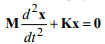where x is a time dependent vector that describes the motion, and M and K are mass and stiffness matrices. Since we are interested in finding harmonic solutions for x, we can simply assume that the solution has the form Xsinωt , and substitute into the equation of motion

-MXω2 sinω t + KX sinωt = 0 ⇒ KX = ω2MX

The vectors u and scalars λ that satisfy a matrix equation of the form Ku  = λMu are called ‘generalized eigenvectors’ and ‘generalized eigenvalues’ of the equation. It is impossible to find exact formulas for λ and u for a large matrix (formulas exist for up to 5x5 matrices, but they are so messy they are useless), but MATLAB has built-in functions that will compute generalized eigenvectors and eigenvalues given numerical values for M and K.

The special values of λ satisfying KX = λMX are related to the natural frequencies by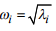The special vectors X are the ‘Mode shapes’ of the system. These are the special initial displacements that will cause the mass to vibrate harmonically.

If you only want to know the natural frequencies (common) you can use the MATLAB command

d = eig(K,M)

This returns a vector d, containing all the values of λ satisfying Ku = λMu  (for an nxn matrix, there are usually n different values). The natural frequencies follow asIf you want to find both the eigenvalues and eigenvectors, you must use

[V,D] = eig(K,M)
This returns two matrices, V and D. Each column of the matrix V corresponds to a vector u that satisfies the equation, and the diagonal elements of D contain the corresponding value of λ . To extract the ith frequency and mode shape, use

omega = sqrt(D(i,i))
X = V(:,i)

For example, here is a MATLAB function that uses this function to automatically compute the natural frequencies of the spring-mass system shown in the figure.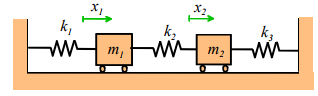function [freqs,modes] = compute_frequencies(k1,k2,k3,m1,m2)

M = [m1,0;0,m2];
K = [k1+k2,-k2;-k2,k2+k3];
[V,D] = eig(K,M);
for i = 1:2
freqs(i) = sqrt(D(i,i));
end modes = V;

end

You could try running this with

>> [freqs,modes] = compute_frequencies(2,1,1,1,1)

This gives the natural frequencies as ω1 = 1, ω2 = 2.236, and the mode shapes as X1 = (-0.707,-0,707) (i.e. both masses displace in the same direction) and X2 = (-0.707,0.707) (the two masses displace in opposite directions.

If you read textbooks on vibrations, you will find that they may give different formulas for the natural frequencies and vibration modes. (If you read a lot of textbooks on vibrations there is probably something seriously wrong with your social life). This is partly because solving Ku = λMu for λ and u is rather complicated (especially if you have to do the calculation by hand), and partly because this formula hides some subtle mathematical features of the equations of motion for vibrating systems. For example, the solutions to Ku = λMu are generally complex (λ and u have real and imaginary parts), so it is not obvious that our guess Xsinωt actually satisfies the equation of motion. It turns out, however, that the equations of motion for a vibrating system can always be arranged so that M and K are symmetric. In this case λ and u are real, and λ is always positive or zero. The old fashioned formulas for natural frequencies and vibration modes show this more clearly. But our approach gives the same answer, and can also be generalized rather easily to solve damped systems (see Section 5.5.5), whereas the traditional textbook methods cannot.

5.5.3 Free vibration of undamped linear systems with many degrees of freedom.

As an example, consider a system with n identical masses with mass m, connected by springs with stiffness k, as shown in the picture. Suppose that at time t=0 the masses are displaced from their static equilibrium position by distances u1,u2..un, and have initial speeds v1,v2... vn. We would like to calculate the motion of each mass x1(t),x2(t)...xn(t) as a function of time.It is convenient to represent the initial displacement and velocity as n dimensional vectors u and v, as u = [u1,u2..un], and v = [v1,v2...vn]. In addition, we must calculate the natural frequencies ωi and mode shapes Xi , i=1..n for the system. The motion can then be calculated using the following formula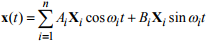where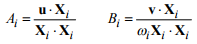Here, the dot represents an n dimensional dot product (to evaluate it in matlab, just use the dot() command).

This expression tells us that the general vibration of the system consists of a sum of all the vibration modes, (which all vibrate at their own discrete frequencies). You can control how big the contribution is from each mode by starting the system with different initial conditions. The mode shapes Xi have the curious property that the dot product of two different mode shapes is always zero ( X1 . X2 = 0 X1 . X3 = 0 , etc) - so you can see that if the initial displacements u happen to be the same as a mode shape, the vibration will be harmonic.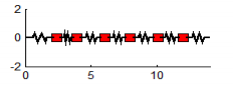The figure on the right animates the motion of a system with 6 masses, which is set in motion by displacing the leftmost mass and releasing it. The graph shows the displacement of the leftmost mass as a function of time. You can download the MATLAB code for this computation here, and see how the formulas listed in this section are used to compute the motion. The program will predict the motion of a system with an arbitrary number of masses, and since you can easily edit the code to type in a different mass and stiffness matrix, it effectively solves any transient vibration problem.

5.5.4 Forced vibration of lightly damped linear systems with many degrees of freedom.

It is quite simple to find a formula for the motion of an undamped system subjected to time varying forces. The predictions are a bit unsatisfactory, however, because their vibration of an undamped system always depends on the initial conditions. In a real system, damping makes the steady-state response independent of the initial conditions. However, we can get an approximate solution for lightly damped systems by finding the solution for an undamped system, and then neglecting the part of the solution that depends on initial conditions.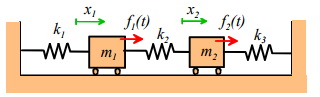As an example, we will consider the system with two springs and masses shown in the picture. Each mass is subjected to a harmonic force, which vibrates with some frequency ω (the forces acting on the different masses all vibrate at the same frequency). The equations of motion are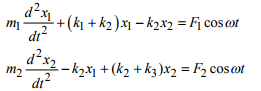We can write these in matrix form as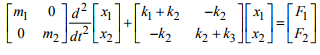or, more generally,To find the steady-state solution, we simply assume that the masses will all vibrate harmonically at the same frequency as the forces. This means that x1 = X1 cosωt , x2 = X2 cosωt , where X1, X2 are the (unknown) amplitudes of vibration of the two masses. In vector form we could write x(t) = X cosωt , where X = [X1, X2] . Substituting this into the equation of motion gives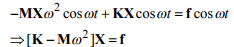This is a system of linear equations for X. They can easily be solved using MATLAB. As an example, here is a simple MATLAB function that will calculate the vibration amplitude for a linear system with many degrees of freedom, given the stiffness and mass matrices, and the vector of forces f.

function X = forced_vibration(K,M,f,omega)
% Function to calculate steady state amplitude of
% a forced linear system.
% K is nxn the stiffness matrix
% M is the nxn mass matrix
% f is the n dimensional force vector
% omega is the forcing frequency, in radians/sec.
% The function computes a vector X, giving the amplitude of
% each degree of freedom
%
X = (K-M*omega^2)\f;
end

The function is only one line long!

As an example, the graph below shows the predicted steady-state vibration amplitude for the spring-mass system, for the special case where the masses are all equal m1 = m2 = m , and the springs all have the same stiffness k1 = k2 = k3 = k . The first mass is subjected to a harmonic force f1 (t) = F1 cosωt , and no force acts on the second mass. Note that the graph shows the magnitude of the vibration amplitude  the formula predicts that for some frequencies some masses have negative vibration amplitudes, but the negative sign has been ignored, as the negative sign just means that the mass vibrates out of phase with the force.Several features of the result are worth noting:

If the forcing frequency is close to any one of the natural frequencies of the system, huge vibration amplitudes occur. This phenomenon is known as resonance. You can check the natural frequencies of the system using the little matlab code in section 5.5.2 – they turn out to be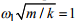and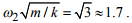At these frequencies the vibration amplitude is theoretically infinite.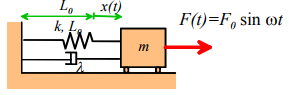The figure predicts an intriguing new phenomenon – at a magic frequency, the amplitude of vibration of mass 1 (that’s the mass that the force acts on) drops to zero. This is called ‘Anti-resonance,’ and it has an important engineering application. Suppose that we have designed a system with a serious vibration problem Usually, this occurs because some kind of unexpected force is exciting one of the vibration modes in the system. We can idealize this behavior as a mass-spring system subjected to a force, as shown in the figure. So how do we stop the system from vibrating? Our solution for a 2DOF system shows that a system with two masses will have an antiresonance. So we simply turn our 1DOF system into a 2DOF system by adding another spring and a mass, and tune the stiffness and mass of the new elements so that the anti-resonance occurs at the appropriate frequency. Of course, adding a mass will create a new vibration mode, but we can make sure that the new natural frequency is not at a bad frequency. We can also add a dashpot in parallel with the spring, if we want – this has the effect of making the anti-resonance phenomenon somewhat less effective (the vibration amplitude will be small, but finite, at the ‘magic’ frequency), but the new vibration modes will also have lower amplitudes at resonance.

Offer running on EduRev: Apply code STAYHOME200 to get INR 200 off on our premium plan EduRev Infinity!

## Introduction to Dynamics and Vibrations- Notes, Videos, MCQs

20 videos|53 docs

,

,

,

,

,

,

,

,

,

,

,

,

,

,

,

,

,

,

,

,

,

;